Interpolation and Extrapolation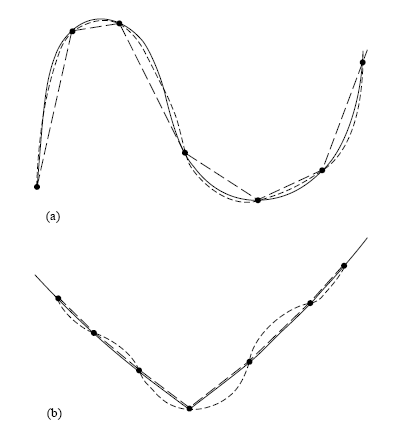(y - y1) / (x - x1) = (y2 - y1) / (x2 -x1)

y = [ (y2 -y1) / (x2 - x1) ] (x - x1) + y1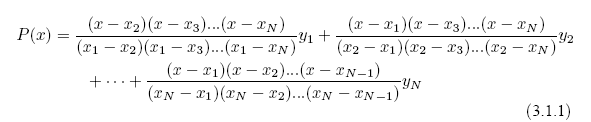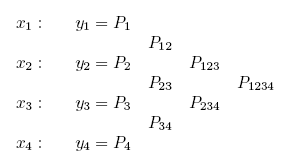Neville's algorithm 厲害的地方，是發現由低階多項式，可以經由組合而系統化地得出更高階多項式（例如自 P12 及 P23 得出 P123），根據下列關係式：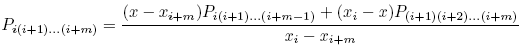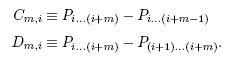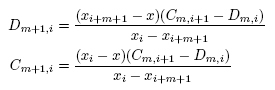call polint( xx(15), yy(15), 4, x, y, dy )

call polint( xx, yy, 18, x, y, dy )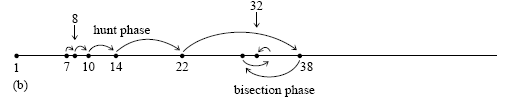k = min( max(j-(m-1)/2,1) , N+1-m )

call polint( xx(k), yy(k), m, x, y, dy )

(1) 注意 polinthunt 或與 locate 的配合。

(2) 使用 polint 時，注意該副程式內己自設上限 NMAX = 10 是最大可用的 n

SUBROUTINE polint(xa,ya,n,x,y,dy)
INTEGER n,NMAX
REAL dy,x,y,xa(n),ya(n)
PARAMETER (NMAX=10) Largest anticipated value of n.

Given arrays xa and ya, each of length n, and given a value x, this routine returns a
value y, and an error estimate dy. If P(x) is the polynomial of degree N ? 1 such that
P(xai) = yai, i = 1, . . . , n, then the returned value y = P(x).

INTEGER i,m,ns
REAL den,dif,dift,ho,hp,w,c(NMAX),d(NMAX)

(3) 要具備使用插值法並呼叫 pgplot 副程式作圖的能力。

program polint_main

c In subroutine polint, array declaration is used for xa(n) and ya(n),
c for this we need to reclare larger or equal size xa(n) and ya(n) in
c this main program. As for NMAX and c(NAMX) and d(NAMX) in that routine,
c they are taken care locally in that routine alreay with specific size
c (parameter) given when declaring array, therefore we don't need to deal
c with that.

implicit none
integer max_tabu, ngrids_max, n_tabu, m, i, j, k, choice
integer k1, k2, more_points, ngrids
parameter (max_tabu = 100, ngrids_max = 2000)
real xa(max_tabu), ya(max_tabu), x, y, dy, delta_x
real x_plot(ngrids_max), y_plot(ngrids_max),dy_plot(ngrids_max)
real x_min, x_max
character*40 filename1

c Ask filename and the number of tabulated points in the file.

write(*,*) 'Type in the file name of tabulated values (x,y)'
write(*,*) 'How many points are there in the tabulated set ?'

if (n_tabu.gt.max_tabu) stop 'Too many tabulated values,
& please recompile the main program with a larger max_tabu.'

c Open the file and read in the tabulated values, and write to screen
c for double check.

open (unit = 20, file = filename1)

do i = 1, n_tabu
write(*,*) xa(i), ya(i)
end do

close (20)

c Ask the order of polynomial to be usedfor interpolation, not higher
c than 10.

1000 continue

write(*,*) 'How many points do you want to form polynomial ?'
write(*,*) 'For eample, 2 points mean linear interpolation.'

if (m.gt.10) then
write(*,*) 'Waring : Neumarial Recipe default max is 10.'
write(*,*) 'in the subroutine polint.'
write(*,*) 'Your choice (0 : exit) or (1: input again).'
if (choice.eq.0) stop 'Terminated by user.'
if (choice.eq.1) goto 1000
goto 1000
endif

c One can choose to type in x one by one or to generte a continuous set
c for graph plotting.

write(*,*) 'What do you want to do ?'
write(*,*)'0:input x, find y ; 1:generate a set of points (x,y)'

c This part get x one by one, using "locate" to find the right interval.

if (choice.eq.0) then

1002 write(*,*) 'Type in your x:'

call locate (xa, n_tabu, x, j)
k = min( max(j-(m-1)/2,1) , n_tabu+1-m )
call polint(xa(k), ya(k), m, x, y, dy)
write(*,*) 'Interpolated value is ', y, ', error is ', dy
write(*,*) 'One more point (yes=1;no=0) ?'
if (more_points.eq.1) goto 1002
endif

c This part generate a set of x, using "hunt" to find the right interval.
c The resulting (x,y) is written out into a file named by user.

if (choice.eq.1) then

write(*,*) 'Type your range x_initial and x-final:'
write(*,*) 'How many points do you want to plot ?'
if(ngrids.le.1) stop 'Need at least two grids.'

delta_x = ( x_max - x_min ) / (ngrids - 1)
j = 1
do i=1, ngrids
x = x_min + (i-1)*delta_x
call hunt(xa, n_tabu, x, j)
k = min( max(j-(m-1)/2,1) , n_tabu+1-m )
x_plot(i) = x
write(*,*) 'x is ',x_plot(i),' j is ',j,' k is',k
call polint(xa(k),ya(k),m,x_plot(i),y_plot(i),dy_plot(i))
end do

write(*,*) 'Plrease give a name to your output file:'
open (unit=30, file=filename1)
do i=1,ngrids
write(30,*) x_plot(i), y_plot(i)
end do
close(30)

write(*,*) 'Info: Error estimate will be written into file
&error.dat'
open (unit=40, file='error.dat')
do i=1,ngrids
write(40,*) x_plot(i), dy_plot(i)
end do
close(40)

end if

end

program polint_pgplot

integer ntab_max, nplot_max
parameter (ntab_max = 20, nplot_max = 200)
real x_tab(ntab_max), y_tab(ntab_max), x_min, x_max, delta_x
real x_plot(nplot_max), y_plot(nplot_max), dy_plot(nplot_max)
integer ntab, i, nplot, m, j, pgopen
character*40 filename1

write(*,*)'Please tell me the number of tabulated values:'

write(*,*) 'Which filename contains the tabulated value ?'

open(unit=10, file=filename1)
do i=1,ntab
end do
close(10)

write(*,*) 'Type in min and max of x:'

write(*,*) 'How many points to draw ?'

write(*,*) 'How many point to form polynomial ?'

delta_x = (x_max - x_min) / (nplot - 1)

do i=1, nplot
x_plot(i) = x_min + (i-1) * delta_x
call hunt(x_tab,ntab,x_plot(i),j)
k = min( max(j-(m-1)/2,1) , ntab+1-m )
call polint(x_tab(k), y_tab(k), m, x_plot(i), y_plot(i),
& dy_plot(i))
end do

do i=1,nplot,10
write(*,*) 'x_plot and y_plot,', x_plot(i), y_plot(i)
end do

if ( pgopen('?') .le. 0 ) stop
call pgenv(2.0, 6.0, 0.0, 40.0, 0, 1)
call pgline(nplot, x_plot, y_plot)
call pgpt(ntab, x_tab, y_tab, 9)
call pgclos

end